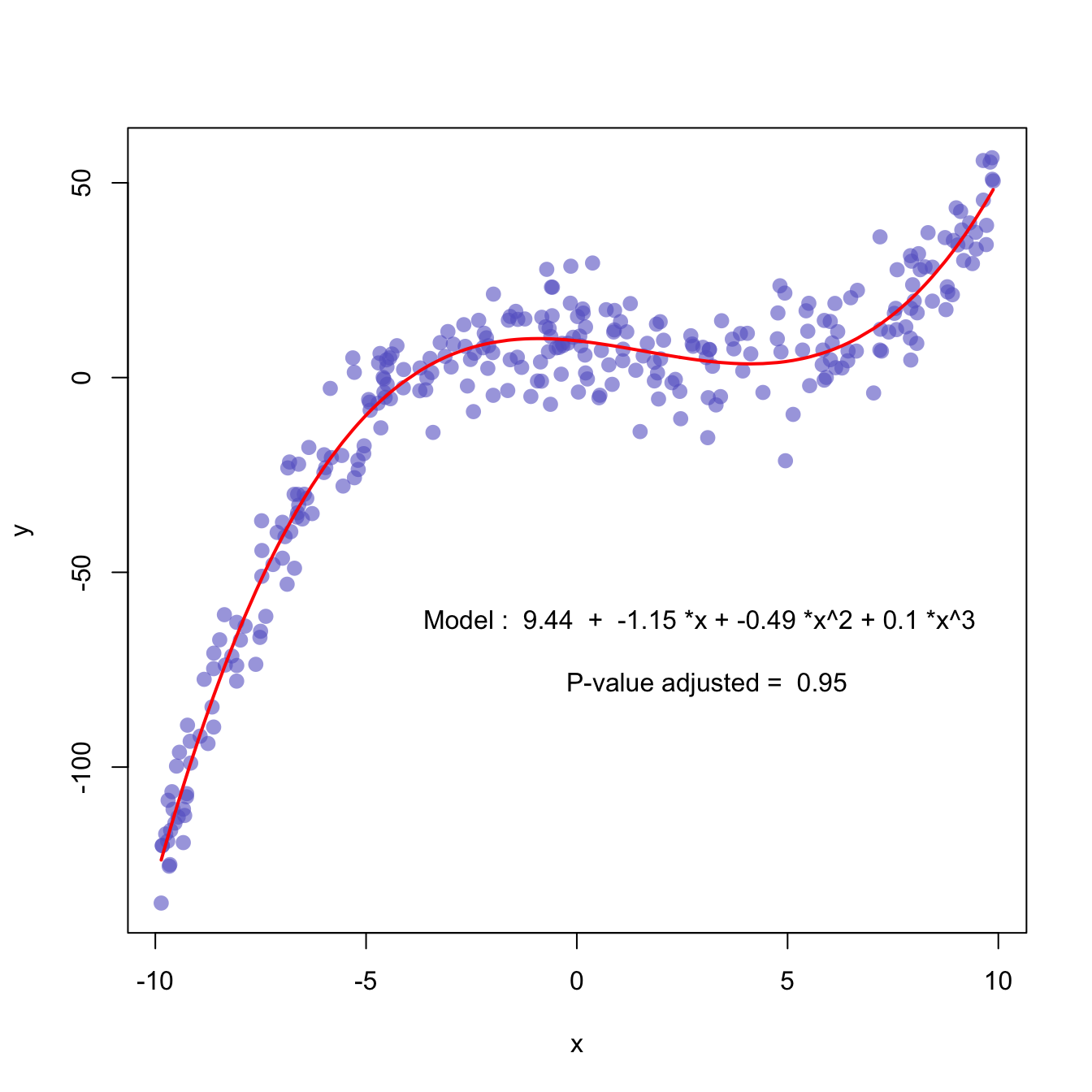# Scatterplot with polynomial curve fitting

This example describes how to build a scatterplot with a polynomial curve drawn on top of it.

First of all, a scatterplot is built using the native R `plot()` function. Then, a polynomial model is fit thanks to the `lm()` function. It is possible to have the estimated Y value for each step of the X axis using the `predict()` function, and plot it with `line()`.

It is a good practice to add the equation of the model with `text()`.

Note: You can also add a confidence interval around the model as described in chart #45.``````x <- runif(300,  min=-10, max=10)
y <- 0.1*x^3 - 0.5 * x^2 - x + 10 + rnorm(length(x),0,8)

# plot of x and y :
plot(x,y,col=rgb(0.4,0.4,0.8,0.6),pch=16 , cex=1.3)

# Can we find a polynome that fit this function ?
model <- lm(y ~ x + I(x^2) + I(x^3))

# I can get the features of this model :
#summary(model)
#model\$coefficients
#summary(model)\$adj.r.squared

# For each value of x, I can get the value of y estimated by the model, and add it to the current plot !
myPredict <- predict( model )
ix <- sort(x,index.return=T)\$ix
lines(x[ix], myPredict[ix], col=2, lwd=2 )

# I add the features of the model to the plot
coeff <- round(model\$coefficients , 2)
text(3, -70 , paste("Model : ",coeff , " + " , coeff , "*x"  , "+" , coeff , "*x^2" , "+" , coeff , "*x^3" , "\n\n" , "P-value adjusted = ",round(summary(model)\$adj.r.squared,2)))``````

Related chart types

## Contact

This document is a work by Yan Holtz. Any feedback is highly encouraged. You can fill an issue on Github, drop me a message on Twitter, or send an email pasting yan.holtz.data with gmail.com.